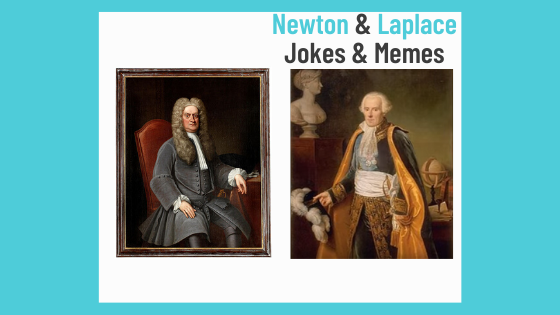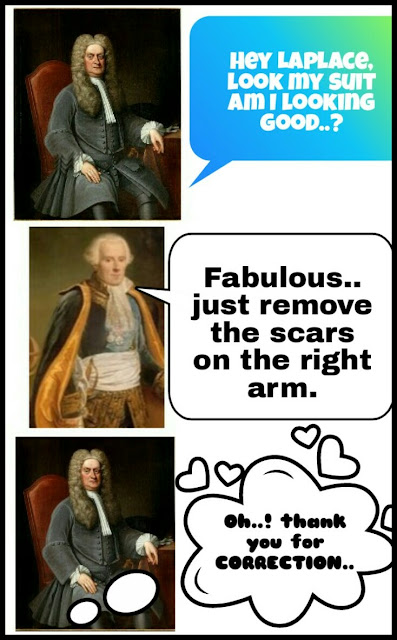# Science Fun and Humor

a blog about science jokes, science memes and science puns with funny description full of science fun and humor. You can find many science memes including chemistry memes, physics memes and jokes, biology memes and jokes and some other fields of science.

## 01 January 2019Newton and Laplace in humorous way; by the Fun and Humor, make the physics fun by understanding the physics humor.by The Fun and Humor

Being so intelligent you must have understood the humor and concept behind this because it is not far more conceptual, and the next thing, that depending upon our personality and nature everyone of us have wide range of thoughts and opinions regarding any topic, meme ,humor, fun or funny material when we try to analyze it in our own personal way at the very 1st look. So i request all of you to make your own ideas at the 1st look of the above image and let your mind to arrange your own humor without reading the below description. After that read the explanation given below and compare your thoughts with that and in the last don't forget to share your ideas because they are so precious for me and i need that..

## EXPLANATION

Newton and Laplace, both of them were the great physicists, everyone is aware of their genius minds. In the 2nd half of 17th century Sir Isaac Newton gave an equation for calculating the speed of sound in any medium. He thought that the travelling of sound through any medium is an isothermal process. So, on the basis of this concept he thought that he predicted the equation accurately but actually he was little bit wrong in calculating the actual speed of sound by his following equation (because he ignored the temperature change in the medium of sound waves).

C =   ˠP/⍴

In the same century another physicist Simon Laplace corrected the flaw in the Newton's equation and added a gamma factor in the equation, on the basis of concept that  the travelling of sound is not an isothermal process. So he corrected the Newton's equation and his work is famous as the  LAPLACE CORRECTION.

Laplace's Equation;

C  =  ˠP/⍴

The gamma factor represents the change in temperature in the medium through which the sound waves travel. By this equation we can calculate the correct speed of sound.

Using this concept i tried to create humor out of the Laplace correction by using the idea of  Newton's suit by my own mind. A scientist don't like the moment when his research or theory is broken or updated by the other scientists. So it is a little bit funny situation when Newton is thanking Laplace for the correction of his suit, but all those readers who already have an idea about Laplace correction can get the humorous point at 1st look. Still the maximum credit goes to the original creator of the equation, Sir Isaac Newton while Laplace has just modified it. Further you yourself are much intelligent that a gesture is more than enough.

Here is another one,now it's easy for u to understand this.by The Fun and Humor.

### LAPLACE CORRECTION IN DETAIL;

You can skip this portion if physics is not your field or if you are not interested in more detail. I am not going in so much depth because my aim is not to share the deep calculations and concepts because they are available in many books and inter net, my aim is to share the fun and humor of science which is not available in the books, that is why a little bit more detail of this topic is given below for those who don't have any idea about this topic and want to understand the humor of the above images..

According to newton the propagation of sound waves is an isothermal process and totally depends upon the density and pressure changes (Elasticity) of the propagating medium.

ⅰ. Density of medium (⍴)
ⅱ. Elasticity of the medium (E)

speed = P/⍴

c= speed of sound,
p= pressure acting on the sound,   and
⍴ = density of the medium.

According to Newton the speed of sound in air is directly proportional to the square root of the pressure of the medium in which the sound is travelling and inversely proportional to the square root of the density of the medium.

By calculating the value of speed by the Newton's formula we get;

v=281 m/s

The experimental value for the speed of sound in gas is 330 m/s, so there is 16% error.

Laplace assumed that sound waves are longitudinal waves which consist of compressions and rarefactions. At the compressions the sound waves are compressed and the temperature of the air rises and at the rarefaction air is cooled down. So the temperature of the gas does not remain same when a sound wave is produced. Therefore Laplace said that Boyle's law is not applicable. Further Laplace also described that air is very poor conductor of heat and electricity due to which the propagation of sound waves through a gas is an adiabatic process. The rapid changes in the air volume, temperature, pressure take place under adiabatic conditions.

Considering above description Laplace gave his equation as;

C =  √ˠ P/⍴

The speed of sound calculated by this formula is 333 m/s which approximately equal to the experimental value within reasonable limits..